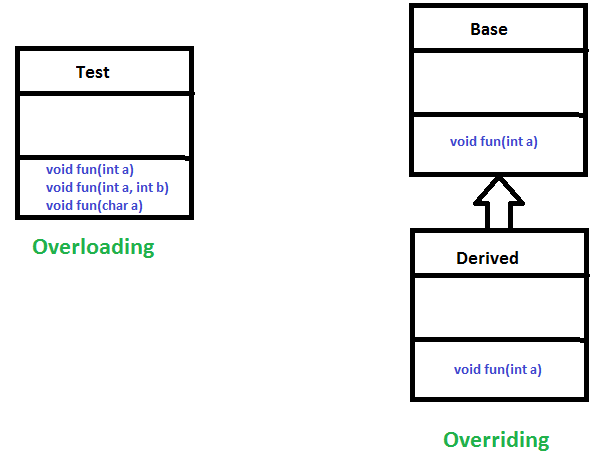# Polymorphism in Java

• Difficulty Level : Easy
• Last Updated : 14 Dec, 2021

The word polymorphism means having many forms. In simple words, we can define polymorphism as the ability of a message to be displayed in more than one form.

Real-life Illustration: Polymorphism

A person at the same time can have different characteristics. Like a man at the same time is a father, a husband, an employee. So the same person possesses different behavior in different situations. This is called polymorphism.
Polymorphism is considered one of the important features of Object-Oriented Programming. Polymorphism allows us to perform a single action in different ways. In other words, polymorphism allows you to define one interface and have multiple implementations. The word “poly” means many and “morphs” means forms, So it means many forms.

Types of polymorphism

In Java polymorphism is mainly divided into two types:

• Compile-time Polymorphism
• Runtime Polymorphism

Type 1: Compile-time polymorphismMethod Overloading: When there are multiple functions with the same name but different parameters then these functions are said to be overloaded. Functions can be overloaded by change in the number of arguments or/and a change in the type of arguments.

Example 1

## Java

 `// Java Program for Method overloading` `// By using Different Types of Arguments `   `// Class 1` `// Helper class` `class` `Helper {`   `    ``// Method with 2 integer parameters` `    ``static` `int` `Multiply(``int` `a, ``int` `b)` `    ``{`   `        ``// Returns product of integer numbers` `        ``return` `a * b;` `    ``}`   `    ``// Method 2` `    ``// With same name but with 2 double parameters` `    ``static` `double` `Multiply(``double` `a, ``double` `b)` `    ``{`   `        ``// Returns product of double numbers` `        ``return` `a * b;` `    ``}` `}`   `// Class 2` `// Main class` `class` `GFG {`   `    ``// Main driver method` `    ``public` `static` `void` `main(String[] args)` `    ``{`   `        ``// Calling method by passing` `        ``// input as in arguments` `        ``System.out.println(Helper.Multiply(``2``, ``4``));` `        ``System.out.println(Helper.Multiply(``5.5``, ``6.3``));` `    ``}` `}`

Output:

```8
34.65```

Example 2

## Java

 `// Java program for Method Overloading` `// by Using Different Numbers of Arguments`   `// Class 1` `// Helper class` `class` `Helper {`   `    ``// Method 1` `    ``// Multiplication of 2 numbers` `    ``static` `int` `Multiply(``int` `a, ``int` `b)` `    ``{`   `        ``// Return product` `        ``return` `a * b;` `    ``}`   `    ``// Method 2` `    ``// // Multiplication of 3 numbers` `    ``static` `int` `Multiply(``int` `a, ``int` `b, ``int` `c)` `    ``{`   `        ``// Return product` `        ``return` `a * b * c;` `    ``}` `}`   `// Class 2` `// Main class` `class` `GFG {`   `    ``// Main driver method` `    ``public` `static` `void` `main(String[] args)` `    ``{`   `        ``// Calling method by passing` `        ``// input as in arguments` `        ``System.out.println(Helper.Multiply(``2``, ``4``));` `        ``System.out.println(Helper.Multiply(``2``, ``7``, ``3``));` `    ``}` `}`

Output:

```8
42```

Type 2: Runtime polymorphism

It is also known as Dynamic Method Dispatch. It is a process in which a function call to the overridden method is resolved at Runtime. This type of polymorphism is achieved by Method Overriding. Method overriding, on the other hand, occurs when a derived class has a definition for one of the member functions of the base class. That base function is said to be overridden.

Example

## Java

 `// Java Program for Method Overriding`   `// Class 1` `// Helper class` `class` `Parent {`   `    ``// Method of parent class` `    ``void` `Print()` `    ``{`   `        ``// Print statement` `        ``System.out.println(``"parent class"``);` `    ``}` `}`   `// Class 2` `// Helper class` `class` `subclass1 ``extends` `Parent {`   `    ``// Method` `    ``void` `Print() { System.out.println(``"subclass1"``); }` `}`   `// Class 3` `// Helper class` `class` `subclass2 ``extends` `Parent {`   `    ``// Method` `    ``void` `Print()` `    ``{`   `        ``// Print statement` `        ``System.out.println(``"subclass2"``);` `    ``}` `}`   `// Class 4` `// Main class` `class` `GFG {`   `    ``// Main driver method` `    ``public` `static` `void` `main(String[] args)` `    ``{`   `        ``// Creating object of class 1` `        ``Parent a;`   `        ``// Now we will be calling print methods` `        ``// inside main() method`   `        ``a = ``new` `subclass1();` `        ``a.Print();`   `        ``a = ``new` `subclass2();` `        ``a.Print();` `    ``}` `}`

Output:

```subclass1
subclass2```

Output explanation:

Here in this program, When an object of child class is created, then the method inside the child class is called. This is because The method in the parent class is overridden by the child class. Since The method is overridden, This method has more priority than the parent method inside the child class. So, the body inside the child class is executed.

My Personal Notes arrow_drop_up
Recommended Articles
Page :